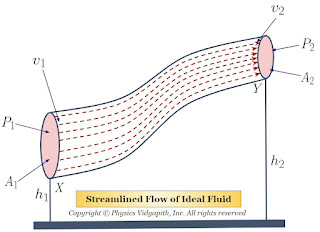## Astrophysics and Astronomy

What is the difference between astrophysics and astronomy?

Astrophysics and astronomy are two closely related fields of study that deal with the study of the universe and its contents, but there are some differences between them.

Astronomy is the study of celestial objects such as stars, planets, galaxies, and other objects that are observed in the universe using telescopes and other observational tools. It deals with the observation and description of these objects, their motions, and interactions.

Astrophysics, on the other hand, is a branch of physics that applies the laws of physics to study the behavior and properties of celestial objects. It uses a combination of theoretical models and observational data to understand the physical processes that occur in the universe, such as the behavior of stars, the evolution of galaxies, the properties of black holes, and the nature of dark matter.

In summary, while astronomy focuses on the observation and description of celestial objects, astrophysics seeks to explain the physical properties and behavior of these objects using the laws of physics. The two fields are closely related and often overlap, with many astronomers also studying astrophysics and vice versa.

## Popular Posts

### Bernoulli's Theorem and Derivation of Bernoulli's EquationStatement of Bernoulli's Theorem: When an ideal fluid (i.e incompressible and non-viscous Liquid or Gas) flows in streamlined motion from one place to another, then the total energy per unit volume (i.e Pressure energy + Kinetic Energy + Potential Energy) at each and every of its path is constant. $P+\frac{1}{2}\rho v^{2} + \rho gh= constant$ Derivation of Bernoulli's Theorem Equation: Let us consider that an incompressible and non-viscous liquid is flowing in streamlined motion through a tube $XY$ of the non-uniform cross-section. Now Consider: The Area of cross-section $X$ = $A_{1}$ The Area of cross-section $Y$ = $A_{2}$ The velocity per second (i.e. equal to distance) of fluid at cross-section $X$ = $v_{1}$ The velocity per second (i.e. equal to distance) of fluid at cross-section $Y$ = $v_{2}$ The Pressure of fluid at cross-section $X$ = $P_{1}$ The Pressure of fluid at cross-section $Y$ = $P_{2}$ The height of cross-section $X$ from